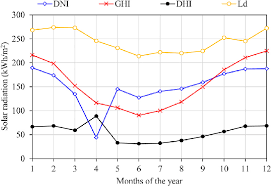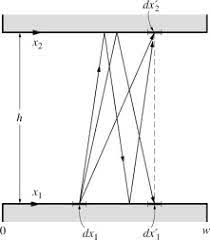## How to Calculate and Solve for Total Irradiation | Radiation Heat TransferThe image above represents total irradiation.

To compute for total irradiation, three essential parameters are needed and these parameters are Reflectivity (ρ), Emissive Power of First Body (e) and Emissive Power of Second Body (e2).

The formula for calculating total irradiation:

G = e2 + ρe / 1 – ρ

Where:

ρ = Reflectivity
e = Emissive Power of First Body
e2 = Emissive Power of Second Body

Let’s solve an example;
Given that the reflectivity is 21, the emissive power of first body is 3 and the emissive power of second body is 9. Find the total irradiation?

This implies that;

ρ = Reflectivity = 21
e = Emissive Power of First Body = 3
e2 = Emissive Power of Second Body = 9

G = e2 + ρe / 1 – ρ
G = 9 + 21(3) / 1 – 21
G = 9 + 63 / -20
G = 72 / -20
G = -3.6

Therefore, the total irradiation is -3.6.

## How to Calculate and Solve for Radiosity | Radiation Heat TransferTo compute for radiosity, three essential parameters are needed and these parameters are Total Emissive Power (e), Reflectivity (ρ) and Total Irradiation (G).

J = e + ρG

Where:

e = Total Emissive Power
ρ = Reflectivity

Let’s solve an example;
Find the radiation heat transfer when the total emissive power is 12, the reflectivity is 10 and the total irradiation is 20.

This implies that;

e = Total Emissive Power = 12
ρ = Reflectivity = 10
G = Total Irradiation = 20

J = e + ρG
J = 12 + (10)(20)
J = 12 + 200
J = 212

Therefore, the radiosity is 212 W/m².

Calculating the Total Emissive Power when the Radiosity, the Reflectivity and the Total Irradiation is Given.

e = J – ρG

Where:

e = Total Emissive Power
ρ = Reflectivity

Let’s solve an example;
Find the total emissive power when the radiation heat transfer is 32, the reflectivity is 10 and the total irradiation is 2.

This implies that;

J = Radiation Heat Transfer = 32
ρ = Reflectivity = 10
G = Total Irradiation = 2

e = J – ρG
e = 32 – (10)(2)
e = 32 – 20
e = 12

Therefore, the total emissive power is 12.